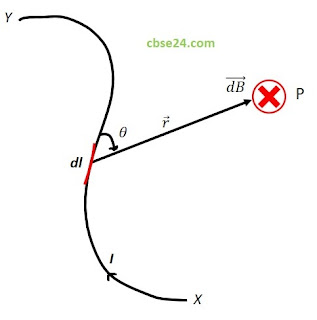# Define Biot-Savart law class 12 notes -cbse24

Oersted experiment showed that a current-carrying conductor produces a magnetic field around it.

A current element denoted by dl, which has the same direction as that of current I. From a series of experiments on current-carrying conductors of simple shapes, two French physicists Jean Baptiste Biot and Felix Savart in 1820, deduced an expression for the magnetic field of a current element which is known as Biot-Savart Law.

Statement:-

Consider a current element dl of a conductor xy carrying I. Let P be the point where the magnetic field due to the current element dl is to be calculated.Biot-Savart law

According to Biot-Savart law, the magnitude of the field dB is

Combining all other four factors we got

Note:-Proportionality constant K depends on the medium between the observation point P and the current element and the system of the unit chosen, for free space  SI units is

Here μo is a constant called the permeability of free space. So the Biot-Savart law expressed as

We can write the above equation as

### Special Cases

• If θ=0,sinθ=0, so that dB=0 i.e., the magnetic field is zero at the point on the axis of the current element.
• If θ=90,sinθ=1, so that dB is maximum i.e. the magnetic field due to a current element is maximum in a plane passing through the element and perpendicular to its axis.

*